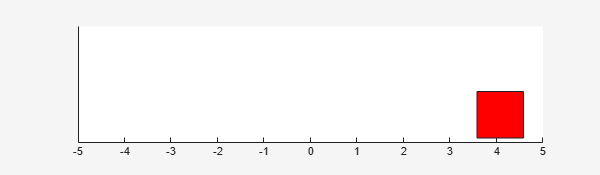# rlPredefinedEnv

Create a predefined reinforcement learning environment

Since R2019a

## Syntax

``env = rlPredefinedEnv(keyword)``

## Description

example

````env = rlPredefinedEnv(keyword)` takes a predefined keyword `keyword` representing the environment name to create a MATLAB® or Simulink® reinforcement learning environment `env`. The environment `env` models the dynamics with which the agent interacts, generating rewards and observations in response to agent actions.```

## Examples

collapse all

Use the predefined `"BasicGridWorld"` keyword to create a basic grid world reinforcement learning environment.

`env = rlPredefinedEnv("BasicGridWorld")`
```env = rlMDPEnv with properties: Model: [1x1 rl.env.GridWorld] ResetFcn: [] ```

Use the predefined `"DoubleIntegrator-Continuous"` keyword to create a continuous double integrator reinforcement learning environment.

`env = rlPredefinedEnv("DoubleIntegrator-Continuous")`
```env = DoubleIntegratorContinuousAction with properties: Gain: 1 Ts: 0.1000 MaxDistance: 5 GoalThreshold: 0.0100 Q: [2x2 double] R: 0.0100 MaxForce: Inf State: [2x1 double] ```

You can visualize the environment using the `plot` function and interact with it using the `reset` and `step` functions.

```plot(env) observation = reset(env)```
```observation = 2×1 4 0 ```
`[observation,reward,isDone] = step(env,16)````observation = 2×1 4.0800 1.6000 ```
```reward = -16.5559 ```
```isDone = logical 0 ```

Use the predefined `"SimplePendulumModel-Continuous"` keyword to create a continuous simple pendulum model reinforcement learning environment.

`env = rlPredefinedEnv("SimplePendulumModel-Continuous")`
```env = SimulinkEnvWithAgent with properties: Model : rlSimplePendulumModel AgentBlock : rlSimplePendulumModel/RL Agent ResetFcn : [] UseFastRestart : on ```

## Input Arguments

collapse all

Predefined keyword representing the environment name, specified as one of the following:

MATLAB Environment

• `'BasicGridWorld'`

• `'CartPole-Discrete'`

• `'CartPole-Continuous'`

• `'DoubleIntegrator-Discrete'`

• `'DoubleIntegrator-Continuous'`

• `'SimplePendulumWithImage-Discrete'`

• `'SimplePendulumWithImage-Continuous'`

• `'WaterFallGridWorld-Stochastic'`

• `'WaterFallGridWorld-Deterministic'`

• `'SimplePendulumModel-Discrete'`

• `'SimplePendulumModel-Continuous'`

• `'CartPoleSimscapeModel-Discrete'`

• `'CartPoleSimscapeModel-Continuous'`

## Output Arguments

collapse all

MATLAB or Simulink environment object, returned as one of the following:

• `rlMDPEnv` object, when you use one of the following keywords.

• `'BasicGridWorld'`

• `'WaterFallGridWorld-Stochastic'`

• `'WaterFallGridWorld-Deterministic'`

• `CartPoleDiscreteAction` object, when you use the `'CartPole-Discrete'` keyword.

• `CartPoleContinuousAction` object, when you use the `'CartPole-Continuous'` keyword.

• `DoubleIntegratorDiscreteAction` object, when you use the `'DoubleIntegrator-Discrete'` keyword.

• `DoubleIntegratorContinuousAction` object, when you use the `'DoubleIntegrator-Continuous'` keyword.

• `SimplePendlumWithImageDiscreteAction` object, when you use the `'SimplePendulumWithImage-Discrete'` keyword.

• `SimplePendlumWithImageContinuousAction` object, when you use the `'SimplePendulumWithImage-Continuous'` keyword.

• `SimulinkEnvWithAgent` object, when you use one of the following keywords.

• `'SimplePendulumModel-Discrete'`

• `'SimplePendulumModel-Continuous'`

• `'CartPoleSimscapeModel-Discrete'`

• `'CartPoleSimscapeModel-Continuous'`

## Version History

Introduced in R2019a